Last updated on 5/4/22

## Declare variables and modify their valuesThe goal of a program is to do something with data, or in other words, stuff you put into your program. Your program uses variables to store and manipulate that data. More specifically, a variable is a container used to store a piece of the data that your program needs to work. A username, the number of available tickets left for a flight, whether or not a certain product is in stock — all of this data is stored in variables.

A piece of data placed in a variable is called a value. Using the "box-in-a-warehouse" analogy, different boxes can store different values. For example, you can use one box to store money for ongoing expenditures, and another to save up for a specific occasion, like going on a trip. You can also empty the boxes or change their content, like adding some money or taking some out.

To know what each box is for, you need to label them. With programming, it's the same: you assign a name to your variable. A variable's name should reflect the meaning of its contents like labels on boxes. Here are some general recommendations for creating names:

• Use descriptive names throughout your code
Did you ever fish an old folder out of a box labeled "admin stuff" on the cover? Remember how frustrating that was? Being descriptive and specific in variable names makes life far easier: it makes your code easier to read and to maintain. Instead of  `amount` (or even worse,  `amt`), add some detail:  `quantityInStock`,  `currentBalance`, etc.

• Spell it out
Avoid abbreviation or shortening words when possible; even if a shorter version seems obvious. For example, `annualRevenue` is better than `annualRev`.

• Follow a common naming convention
One of the popular naming conventions is called camel case — names made up of multiple words where all but the first are capitalized e.g. `numberOfCats`,  `finalAnswer`,  `howLongCanThisVariableNameGet`, etc.

To use a variable in code, you must create one. This is called declaring a variable.

In JavaScript, declaring a variable is as simple as using the `let` keyword, followed by the chosen variable name: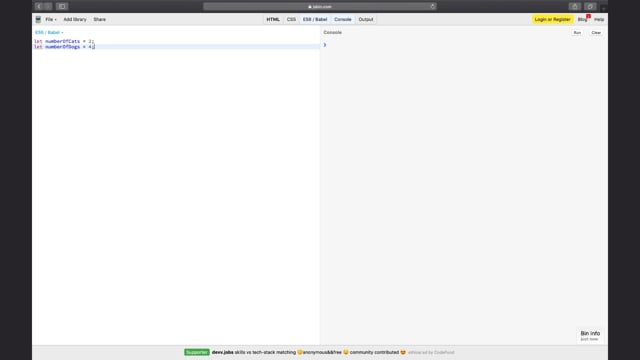``````let numberOfCats = 2;
let numberOfDogs = 4;
``````

Here, we declare (create) and initialize (give a value to) two variables:  `numberOfCats` and  `numberOfDogs`.

#### Practice creating variables!

Head to CodePen Exercise P1CH2a and follow the instructions below to practice creating and initializing two variables.

You will create two variables which are needed by a component built by your colleague. Together, you are building the list view for TV shows, and each TV show needs a variable for the number of seasons that show has, and the number of episodes there are in each season.

Your colleague requests that you name these variables numberOfSeasons and numberOfEpisodes in order to be compatible with their code.

1. Create a variable called numberOfSeasons and assign it the value 6 between the lines created for you in the JavaScript editor (the tab labeled "JS"). Remember to use the let keyword, and don't forget the semicolon at the end of the line!

2. Next, create a variable called numberOfEpisodes, and assign it the value 12.

#### Solution:

Once you've given it a go, watch me code a solution and see how your approach compares. And here is a new CodePen with a solution to the exercice.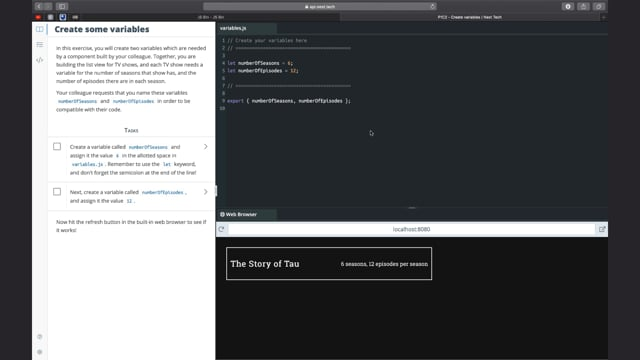The first and easiest way of changing the value of a variable is simply to reassign it: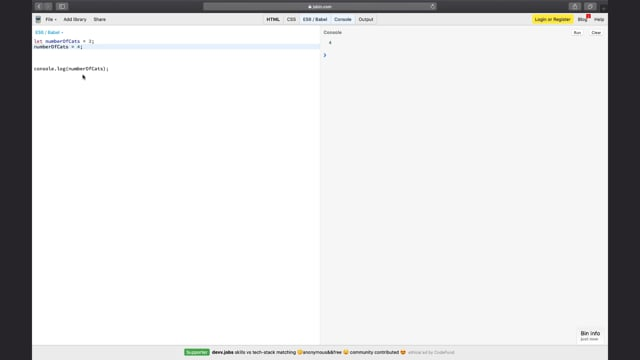``````let numberOfCats = 3;
numberOfCats = 4;
``````

Here, we declare the variable numberOfCats and initialize it with the value 3. We then reassign it the value 4 (without the `let` keyword, as the variable has already been declared).

However, if that were the only way of changing a variable's value, you wouldn't be able to do an awful lot. Let's have a look at some operators.

#### Arithmetic operators - working with numbers

Using arithmetic operators allows you to perform basic mathematical operations like addition, subtraction, multiplication, and division. All of these operations can be extremely useful, even outside of mathematical contexts.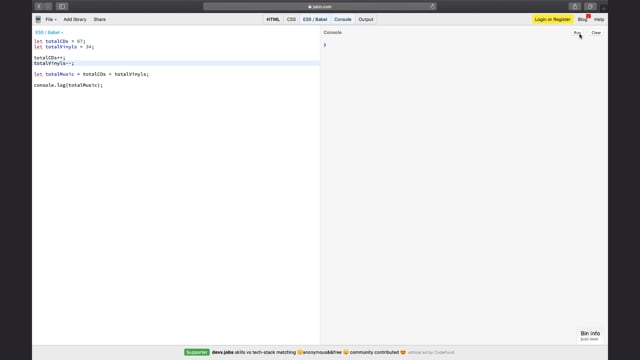To add two variables together, use the `+`  sign:

``````let totalCDs = 67;
let totalVinyls = 34;

let totalMusic = totalCDs + totalVinyls;
``````

Inversely, subtraction uses the `-`  sign:

``````let cookiesInJar = 10;

``````

When adding or subtracting a number from a variable, you can use the  `+=`  and  `-=` operators:

``````let cookiesInJar = 10;

/* bake a new batch of cookies */
cookiesInJar += 12; // there are now 20 cookies in the jar
``````

Finally, you can use  `++`  or  `--`  to add or subtract 1 (increment or decrement):

``````let numberOfLikes = 10;

numberOfLikes++;  // makes 11

numberOfLikes--; // and back to 10...who disliked my post??
``````
##### Multiplication and division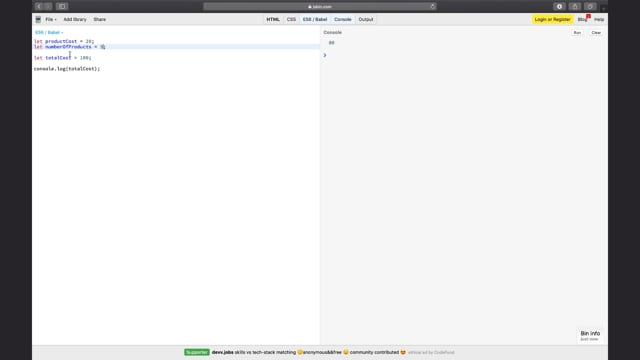Multiplication and division operations use the  `*`  and  `/`  operators:

``````let costPerProduct = 20;
let numberOfProducts = 5;

let totalCost = costPerProduct * numberOfProducts;

let averageCostPerProduct = totalCost / numberOfProducts;
``````

As with addition and subtraction, you have the  `*=`  and  `/=`  operators:

``````let numberOfCats = 2;

numberOfCats *= 6;  // numberOfCats is now 2*6 = 12;

numberOfCats /= 3; // numberOfCats is now 12/3 = 4;
``````

Now it's time for some practice!

#### Practice using operators!

Head to CodePen Exercise P1CH2b and follow the instructions below to practice using operators to modify the value of a variable.

Time to add a new feature to our show preview box: the total viewing time for a show (very important info for binge-watchers!).

1. In the JS editor, create two variables, episodeTime and commercialTime, with appropriate values (45 and 5, for example).

2. Using the two time variables you just created, plus the pre-existing number of episodes and number of seasons variables, create a variable called totalShowTime containing the total viewing time for this show.

The numberOfSeasons and numberOfEpisodes variables have been declared for you in this exercise.

#### Solution:

Once you've given it a go, watch me code a solution and see how your approach compares. And here is a new CodePen with a solution to the exercice.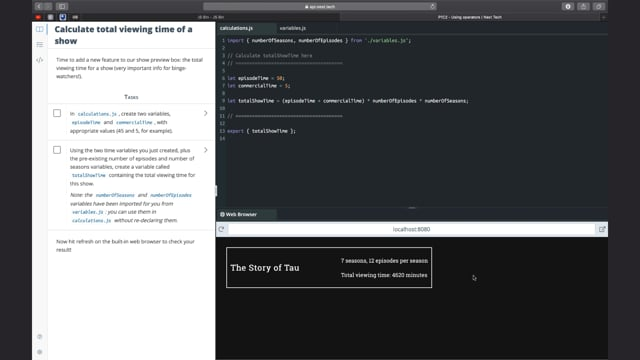There are many other ways of manipulating variables, and you will see a lot of them throughout the rest of this course!

In many programs, some pieces of data will not be modified at any point while the program is running. For example, a company's name, a user's date of birth, or the number of hours in a day. To help make sure you don't accidentally reassign new values to these important pieces of data, you use constants.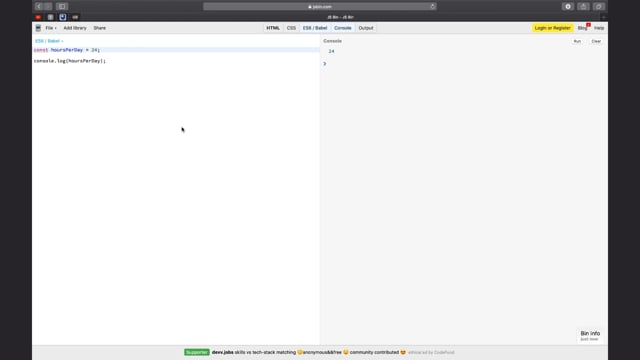#### Practice using constants!

Head to CodePen Exercise P1CH2c and follow the instructions below to practice using constants to manage the value of specific variables.

This is a small component which breaks a number of days into hours, minutes, and seconds. All you have to do is create the three constants which give the number of hours per day, the number of minutes per hour, and the number of seconds per minute. Playing around with these values will show you how important it is to have the correct constant values!

1. In the allotted space, create the following three constants:

• hoursPerDay

• minutesPerHour

• secondsPerMinute

Initialize them with the correct values.

#### Solution:

Once you've given it a go, watch me code a solution and see how your approach compares. And here is a new CodePen with a solution to the exercice.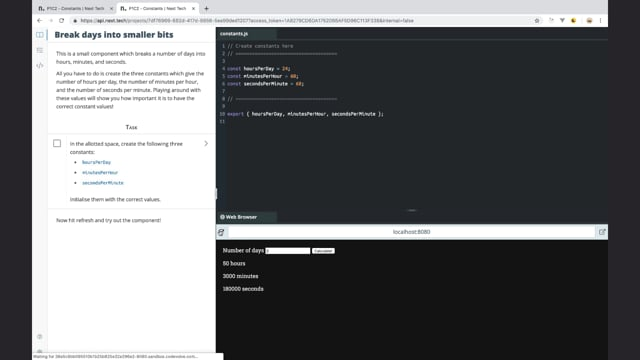In this chapter, you learned the basics of variables in JavaScript.

• Declare variables with the  `let` keyword and a camel case name, and initialize them with the  `=`  operator.

• Modify the contents of a variable by reassigning it or with operators.

• Use constants to prevent essential pieces of data from being over-written.

In the next chapter, we will have a look at an important attribute of variables: their type.